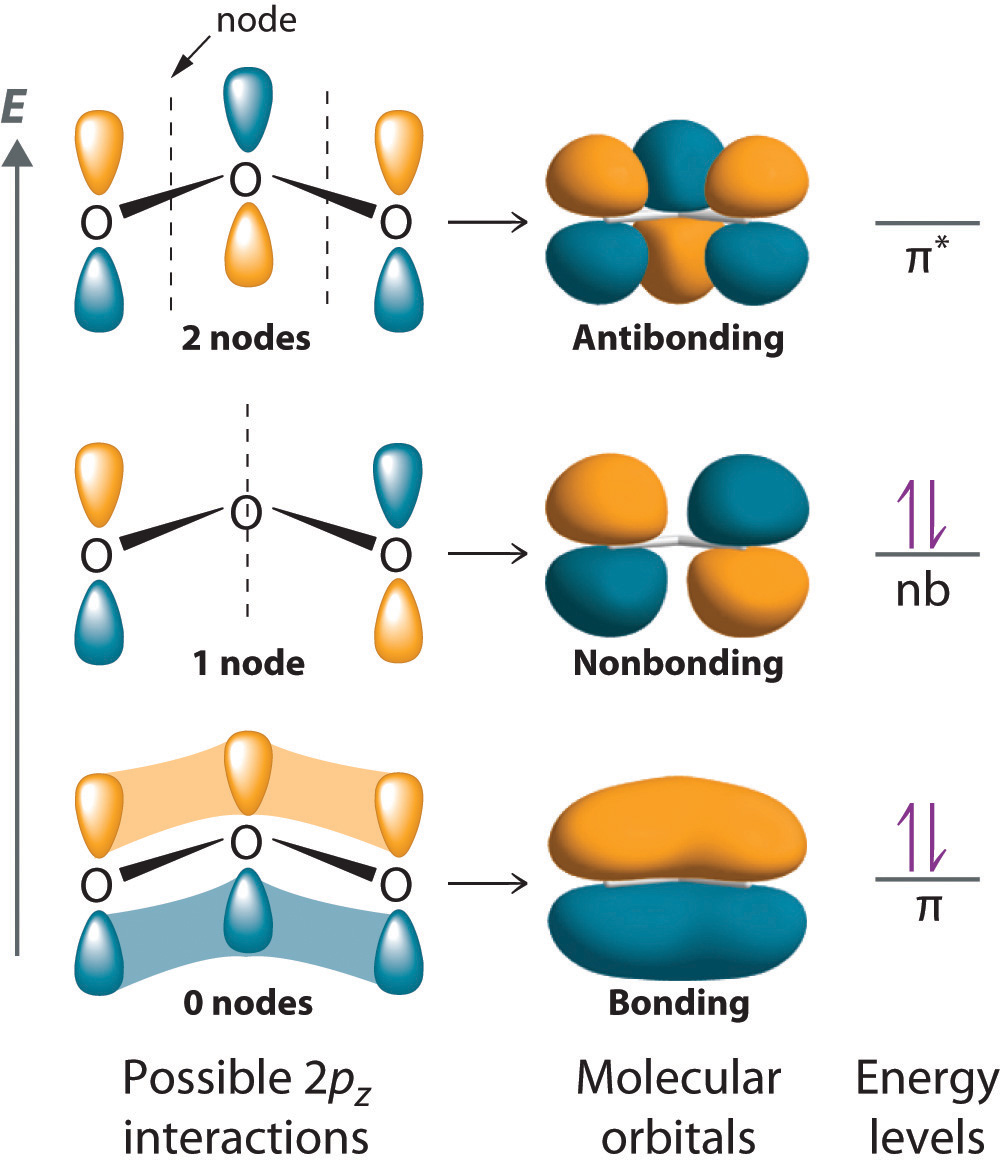# What are nonbonding molecular orbitals?

Apr 13, 2014

A non-bonding orbital (NBMO) is a molecular orbital for which the addition or removal of an electron does not change the energy of the molecule.

Molecular orbitals come from the linear combination of atomic orbitals.

In a simple diatomic molecule such as HF, F has more electrons than H.The s orbital of H can overlap with the $2 {p}_{z}$ orbital of fluorine to form a bonding σ and an antibonding σ* orbital.

The ${p}_{x}$ and ${p}_{y}$ orbitals from the F do not have any other orbitals to combine with. They become NBMOs.

The ${p}_{x}$ and ${p}_{z}$ atomic orbitals have become molecular orbitals. They look like ${p}_{x}$ and ${p}_{y}$ orbitals but they are now molecular orbitals.

The energies of these orbitals are the same in the molecule as they are in an isolated F atom. Thus, putting an electron into them does not change the stability of the molecule.

NBMOs do not need to look like atomic orbitals. For example, the NBMO of the ozone molecule has its electron density concentrated on the end oxygen atoms. There is no electron density on the central atom.